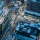Home
IT Knowledge
Inspiration
Languages
EN

# JavaScript - setInterval example

0 points
Created by:p_agon
409

In this article, we would like to show you how to use `setInterval` in JavaScript.

Quick solution:

``````setInterval(() => {
console.log('This will be called every 1000ms (1s)');
}, 1000);``````

`setInterval` is a method that takes in two arguments:

• function which is being called every specific amount of time (interval),
• the interval time (in milliseconds).

`setInterval` is executed until it's stopped by `clearInterval` method which takes the `interval` as an argument.

Below we present some practical examples for better understanding.

## 1. Simple `setInterval` example

In this example, we create and call a simple function that displays some text every second.

Runnable example:

``````// ONLINE-RUNNER:browser;

setInterval(() => {
console.log('This will be called every 1000ms (1s)');
}, 1000);``````

## 2. Calling `setInterval` the specified amount of times

In this example, we create a function with `setInterval` that counts to 5 every second.

Runnable example:

``````// ONLINE-RUNNER:browser;

let x = 0;
let interval = setInterval(() => {
console.log(x + 1);
if (++x === 5) {
clearInterval(interval);
}
}, 1000);``````

## 3. Custom `setInterval`

In this example, we create `customSetInterval` that takes in three arguments:

• `callback` - a function to be called every specific amount of time,
• `delay` - interval time,
• `numberOfTimes` - how many times we want to call the `callback` function.

Runnable example:

``````// ONLINE-RUNNER:browser;

function foo(){
console.log('some text');
return;
};

function customSetInterval(callback, delay, numberOfTimes) {
var x = 0;
var interval = setInterval(() => {
callback();
if (++x === numberOfTimes) {
clearInterval(interval);
}
}, delay);
};

customSetInterval(foo, 1000, 5);``````